Function Repository Resource:

HashHue

Map an expression to a color based on a hash

Contributed by: Bob Sandheinrich
 ResourceFunction["HashHue"][expr] gives a Hue based on the expression expr. ResourceFunction["HashHue"][expr,type] uses the specified hash type.

Details and Options

ResourceFunction["HashHue"] accepts the same values of type as Hash. The default is "Expression".
ResourceFunction["HashHue"] always gives the same Hue for the same input expression.
Coloring complex expressions makes differences stand out much faster than viewing them normally.

Examples

Basic Examples

Get a color for an expression:

 In:=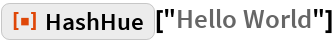Out=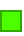Sometime differences in values are hard to see visually:

 In:=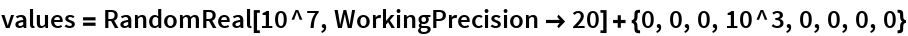Out=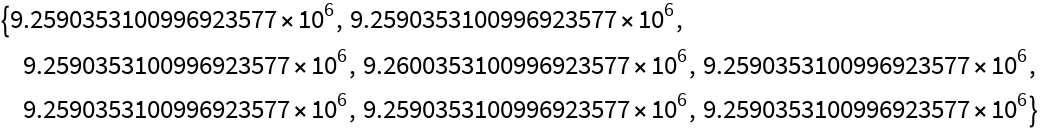Make different values standout visually:

 In:=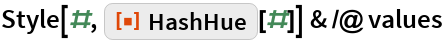Out=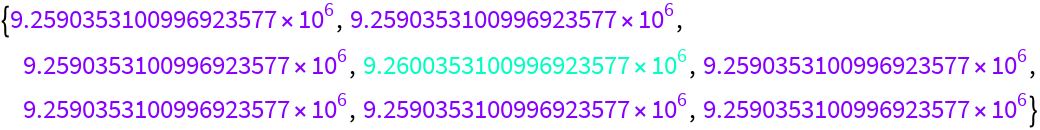Scope

Data with text fields can take up space:

 In:=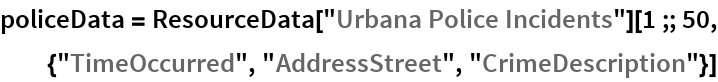Out=Instead, visualize the data with colors where identical values always match:

 In:=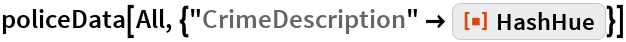Out=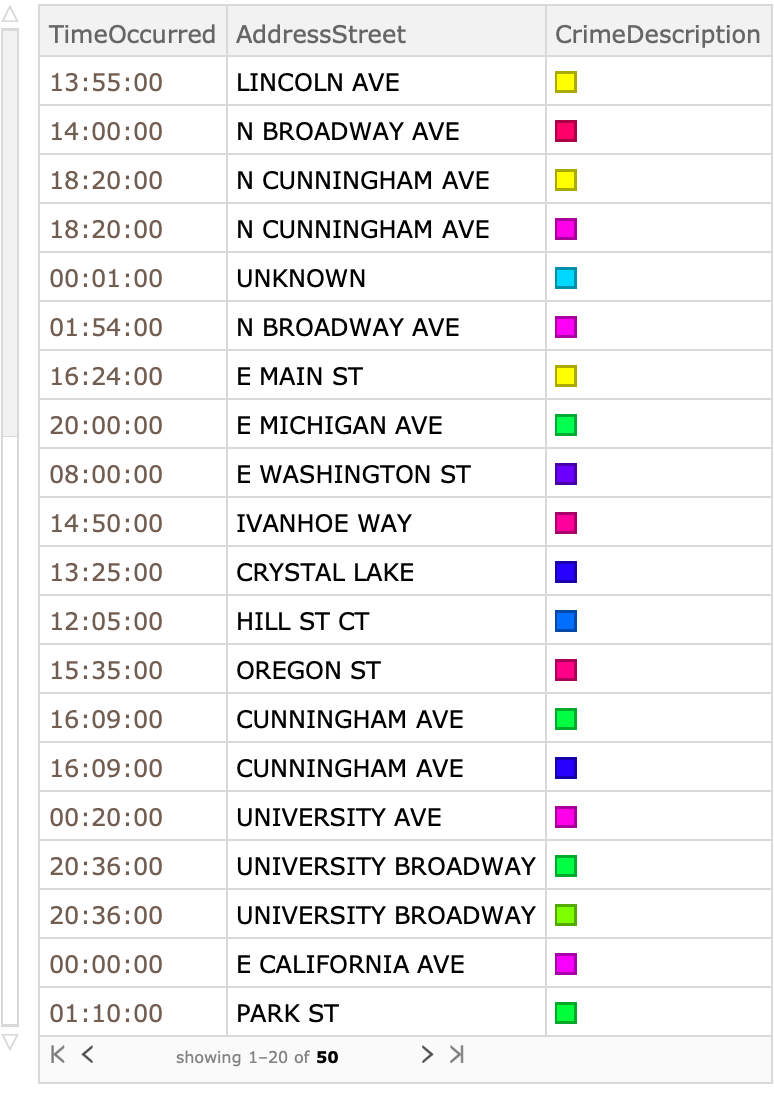Create an index for interpreting the color:

 In:=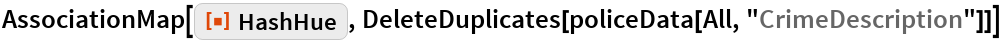Out=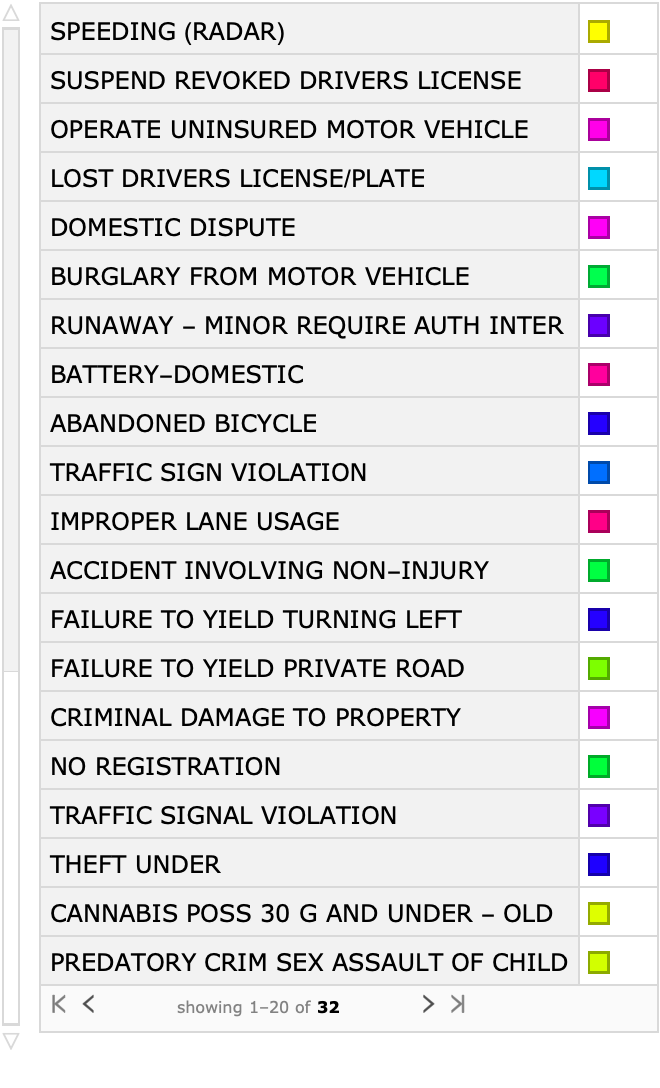Applications

Create a docked cell that shows your current Wolfram Language status:

 In:=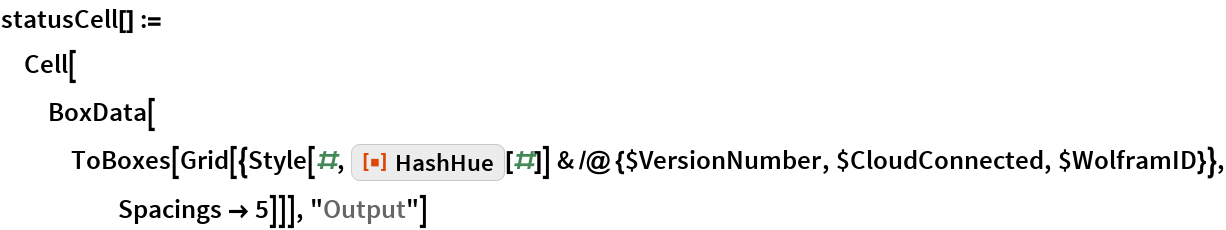In:=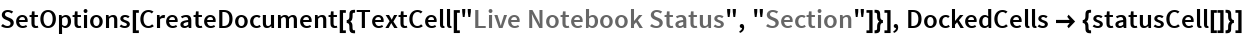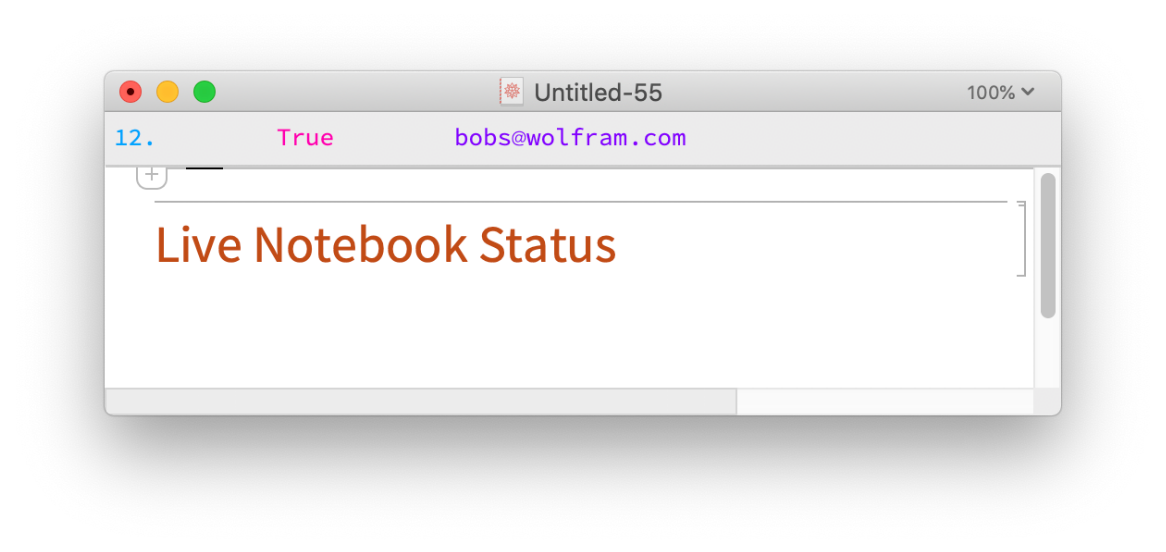Author Notes

Extending this to set all HSB (or RGB) values in a smart way would extend the used color space and increase the typical distance between results.